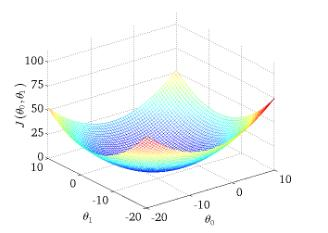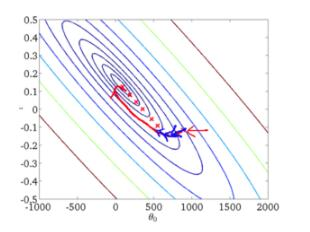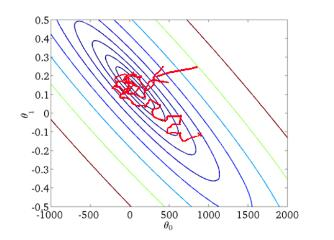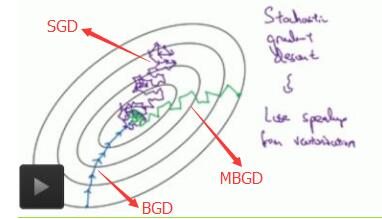# 批量梯度下降(BGD)、随机梯度下降(SGD)以及小批量梯度下降(MBGD)的理解

为了便于理解，这里我们将使用只含有一个特征的线性回归来展开。此时线性回归的假设函数为：

$h_{\theta} (x^{(i)})=\theta_1 x^{(i)}+\theta_0$

其中 $i=1,2,...,m$ 表示样本数。
对应的目标函数（代价函数）即为：

$J(\theta_0, \theta_1) = \frac{1}{2m} \sum_{i=1}^{m}(h_{\theta}(x^{(i)}) - y^{(i)})^2$

下图为 $J(\theta_0,\theta_1)$ 与参数 $\theta_0,\theta_1$ 的关系的图：批量梯度下降法是最原始的形式，它是指在每一次迭代时使用所有样本来进行梯度的更新。从数学上理解如下：
（1）对目标函数求偏导：

$\frac{\Delta J(\theta_0,\theta_1)}{\Delta \theta_j} = \frac{1}{m} \sum_{i=1}^{m} (h_{\theta}(x^{(i)})-y^{(i)})x_j^{(i)}$

其中 $i=1,2,...,m$ 表示样本数， $j = 0,1$ 表示特征数，这里我们使用了偏置项 $x_0^{(i)} = 1$ 。
（2）每次迭代对参数进行更新：

$\theta_j := \theta_j - \alpha \frac{1}{m} \sum_{i=1}^{m} (h_{\theta}(x^{(i)})-y^{(i)})x_j^{(i)}$

注意这里更新时存在一个求和函数，即为对所有样本进行计算处理，可与下文SGD法进行比较。
伪代码形式为：
repeat{
$\theta_j := \theta_j - \alpha \frac{1}{m} \sum_{i=1}^{m} (h_{\theta}(x{(i)})-y{(i)})x_j^{(i)}$
(for j =0,1)
}

优点：
（1）一次迭代是对所有样本进行计算，此时利用矩阵进行操作，实现了并行。
（2）由全数据集确定的方向能够更好地代表样本总体，从而更准确地朝向极值所在的方向。当目标函数为凸函数时，BGD一定能够得到全局最优。
缺点：
（1）当样本数目 $m$ 很大时，每迭代一步都需要对所有样本计算，训练过程会很慢。
从迭代的次数上来看，BGD迭代的次数相对较少。其迭代的收敛曲线示意图可以表示如下：随机梯度下降法不同于批量梯度下降，随机梯度下降是每次迭代使用一个样本来对参数进行更新。使得训练速度加快。
对于一个样本的目标函数为：

$J^{(i)}(\theta_0,\theta_1) = \frac{1}{2}(h_{\theta}(x^{(i)})-y^{(i)})^2$

（1）对目标函数求偏导：

$\frac{\Delta J^{(i)}(\theta_0,\theta_1)}{\theta_j} = (h_{\theta}(x^{(i)})-y^{(i)})x^{(i)}_j$

（2）参数更新：

$\theta_j := \theta_j - \alpha (h_{\theta}(x^{(i)})-y^{(i)})x^{(i)}_j$

注意，这里不再有求和符号
伪代码形式为：
repeat{
for i=1,...,m{
$\theta_j := \theta_j -\alpha (h_{\theta}(x{(i)})-y{(i)})x_j^{(i)}$
(for j =0,1)
}
}

优点：
（1）由于不是在全部训练数据上的损失函数，而是在每轮迭代中，随机优化某一条训练数据上的损失函数，这样每一轮参数的更新速度大大加快。
缺点：
（1）准确度下降。由于即使在目标函数为强凸函数的情况下，SGD仍旧无法做到线性收敛。
（2）可能会收敛到局部最优，由于单个样本并不能代表全体样本的趋势。
（3）不易于并行实现。

解释一下为什么SGD收敛速度比BGD要快：
答：这里我们假设有30W个样本，对于BGD而言，每次迭代需要计算30W个样本才能对参数进行一次更新，需要求得最小值可能需要多次迭代（假设这里是10）；而对于SGD，每次更新参数只需要一个样本，因此若使用这30W个样本进行参数更新，则参数会被更新（迭代）30W次，而这期间，SGD就能保证能够收敛到一个合适的最小值上了。也就是说，在收敛时，BGD计算了 $10 \times 30W$ 次，而SGD只计算了 $1 \times 30W$ 次。

从迭代的次数上来看，SGD迭代的次数较多，在解空间的搜索过程看起来很盲目。其迭代的收敛曲线示意图可以表示如下：小批量梯度下降，是对批量梯度下降以及随机梯度下降的一个折中办法。其思想是：每次迭代 使用 ** batch_size** 个样本来对参数进行更新。
这里我们假设 $batch_size = 10$ ，样本数 $m=1000$ 。
伪代码形式为：
repeat{
for i=1,11,21,31,...,991{
$\theta_j := \theta_j - \alpha \frac{1}{10} \sum_{k=i}{(i+9)}(h_{\theta}(x{(k)})-y{(k)})x_j{(k)}$
(for j =0,1)
}
}

优点：
（1）通过矩阵运算，每次在一个batch上优化神经网络参数并不会比单个数据慢太多。
（2）每次使用一个batch可以大大减小收敛所需要的迭代次数，同时可以使收敛到的结果更加接近梯度下降的效果。(比如上例中的30W，设置batch_size=100时，需要迭代3000次，远小于SGD的30W次)
（3）可实现并行化。
缺点：
（1）batch_size的不当选择可能会带来一些问题。

batcha_size的选择带来的影响：
（1）在合理地范围内，增大batch_size的好处：
a. 内存利用率提高了，大矩阵乘法的并行化效率提高。
b. 跑完一次 epoch（全数据集）所需的迭代次数减少，对于相同数据量的处理速度进一步加快。
c. 在一定范围内，一般来说 Batch_Size 越大，其确定的下降方向越准，引起训练震荡越小。
（2）盲目增大batch_size的坏处：
a. 内存利用率提高了，但是内存容量可能撑不住了。
b. 跑完一次 epoch（全数据集）所需的迭代次数减少，要想达到相同的精度，其所花费的时间大大增加了，从而对参数的修正也就显得更加缓慢。
c. Batch_Size 增大到一定程度，其确定的下降方向已经基本不再变化。

下图显示了三种梯度下降算法的收敛过程：posted @ 2018-08-10 11:57  LLLiuye  阅读(81162)  评论(19编辑  收藏  举报